#### Concept of Practical Logic Characteristics

Practical Logic Characteristics:

In practical logic and switching circuits, the performance is not ideal. Therefore parameters should be established that measure how close the ideal circuit performance is with regard to significant features like speed, noise immunity and drive capability. Some of such parameters are as follows:

a) Logic Voltages: These are defined as voltage levels in the logic family that, if maintained all through, will guarantee accurate operation of the logic circuit.

ViLMAX = maximum voltage acceptable as the logic LO input

ViHMIN = minimum voltage acceptable as the logic HI input.

VOLMAX = maximum voltage acceptable as the logic LO output.

VOHMIN = minimum voltage acceptable as the logic HI output.

Such limiting voltages are shown in figure below. These voltages are stated from the coordinates on input-output transfer characteristic where the slope is –1. It is done on the basis of effect on the circuit which applies to noise and interference components superimposed on the logic signal.

Consider steadily changing the input voltage from one logic state to other. Raising Vi from 0V, the output voltage drops slowly at first till the point at which the slope = -1. Subsequent to this, the output drops at a rapid rate than the input rises. In fast transition region of characteristic in figure shown below, the small signal gain is much bigger than unity. This signifies that any noise superimposed on input as shown in figure below will be amplified and might cause a change of state at the output. In upper part of curve, this doesn’t occur. A similar procedure applies to reducing the input voltage from VCC.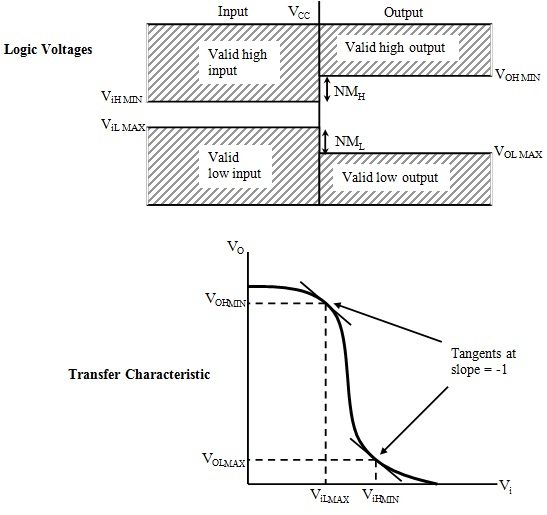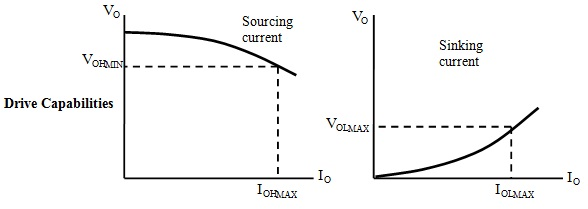Figure: Practical Static Logic Characteristic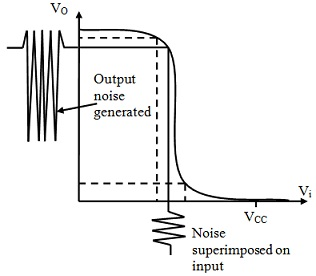Figure: Effect of Noise Superimposed on a Logic Input

b) Noise Immunity: The transfer characteristic shown in figure above is deliberately shaped and hence:

ViH MIN < V OHMIN and ViLMAX > VOL MAX

This permits a margin in the voltage levels and hence an amount of noise can be superimposed on the output voltage of one gate prior to the logic level is misinterpreted at input of another gate that it is driving. This can be seen in figure below. Noise Margins are stated as:

NMH = VOHMIN – ViHMIN and NML = ViLMAX - VOLMAX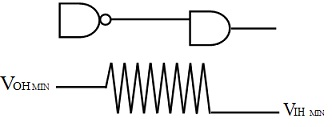Figure: Advantage of allowing a Noise Margin

c) Drive Capability: There is a limit to number of gates which can be joined as load to the output of a single gate that will permit correct voltage levels to be maintained. The current which the driving gate can sink or source is limited and the gate as well has finite output impedance. The output voltage vs. current characteristics is as shown in first figure.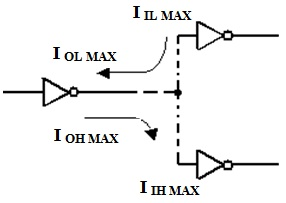Figure: Input and Output Load Currents in Logic Gates

With reference to figure above Fan-out is the maximum number of similar gates which can be joined to a gate output and is stated as:

F = IOHMAX/IiHMAX or IOLMAX/IiLMAX; whichever is lowest

d) Switching Times: The figures of merit employed to characterize the speed of operation of logic gates are its switching times as shown in figure below. The rise and fall times are evaluated between 10% and 90% points on the voltage waveform transitions at gate output. Propagation delays are the delays resultant in a change of state occurring at the output of a gate in response to change of state applied to one of its inputs. These are evaluated between 50% points on the input and output voltage waveforms.

e) Dynamic Noise Immunity: a noise spike, that temporarily brings the input voltage across switching threshold, can frequently be tolerated, devoid of a consequential change of state at output, if there is not sufficient energy in the pulse to cause a change of state. In common, this is the case when the duration of spike is less than the gate propagation delay as shown in figure below.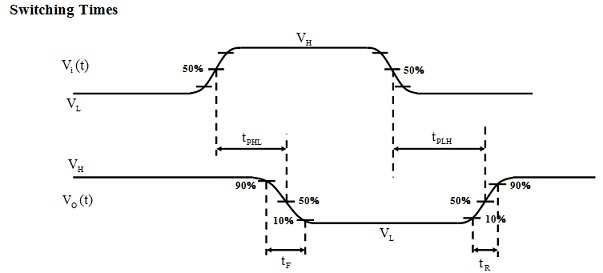tPLH = propagation time for low-to-high output transition

tPHL = propagation time for high-to-low output transition

tR = 10%-to-90% rise time of the output voltage

tF = 90%-to-10% fall time of the output voltage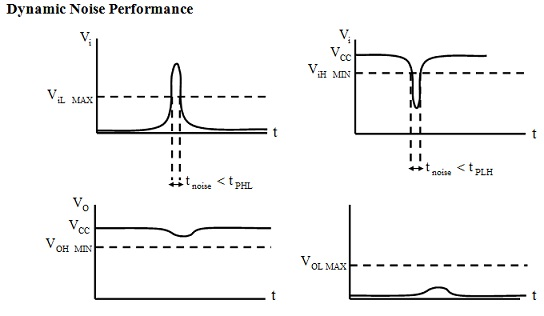Figure: Practical Dynamic Logic Characteristics

Limitations of Single Transistor Switching Circuits:

a) Finite Gain: The limited gain given by a single transistor limits the steepness of the transfer characteristic in transition region between LO and HI output voltages.

b) Logic Voltage Dependence on Load: Whenever the transistor in simple bipolar inverter circuit shown in figure below is OFF, the output Logic HI voltage is as:

VH = [RL/(RL + RC)] VCC

It is heavily dependent on RL. Clearly this condition is totally undesirable and in practice the output voltage must be as independent of the load as possible in the boundaries of operation, that is, the fan-out specification. A means should be found of enhanced defining the output logic HI voltage.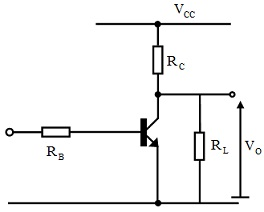Figure: Voltage Dependence on Load of Simple Bipolar Logic Gates

c) Slow Transistor Turn-Off: When Vi → 0V, the transistor in simple bipolar inverter circuit of figure shown below turns OFF. To accomplish this, the overdrive charge stored in base region in saturation should be eliminated. This is done by a small discharge current in base IB = - VBE/RB that makes the procedure quite slow. In addition, any load capacitance present should be charged up via the collector resistor RC that further delays the output transition from logic LO to logic HI. A means of eliminating the charge stored in the base of transistor should be found, and also a means of charging up load capacitance more quickly.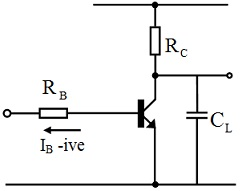Figure: Speed Limitation of Simple Bipolar Logic Gates

Latest technology based Electrical Engineering Online Tutoring Assistance

Tutors, at the www.tutorsglobe.com, take pledge to provide full satisfaction and assurance in Electrical Engineering help via online tutoring. Students are getting 100% satisfaction by online tutors across the globe. Here you can get homework help for Electrical Engineering, project ideas and tutorials. We provide email based Electrical Engineering help. You can join us to ask queries 24x7 with live, experienced and qualified online tutors specialized in Electrical Engineering. Through Online Tutoring, you would be able to complete your homework or assignments at your home. Tutors at the TutorsGlobe are committed to provide the best quality online tutoring assistance for Electrical Engineering Homework help and assignment help services. They use their experience, as they have solved thousands of the Electrical Engineering assignments, which may help you to solve your complex issues of Electrical Engineering. TutorsGlobe assure for the best quality compliance to your homework. Compromise with quality is not in our dictionary. If we feel that we are not able to provide the homework help as per the deadline or given instruction by the student, we refund the money of the student without any delay.